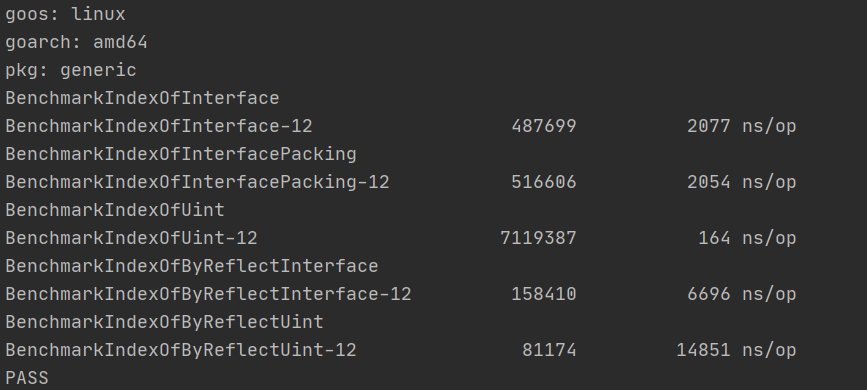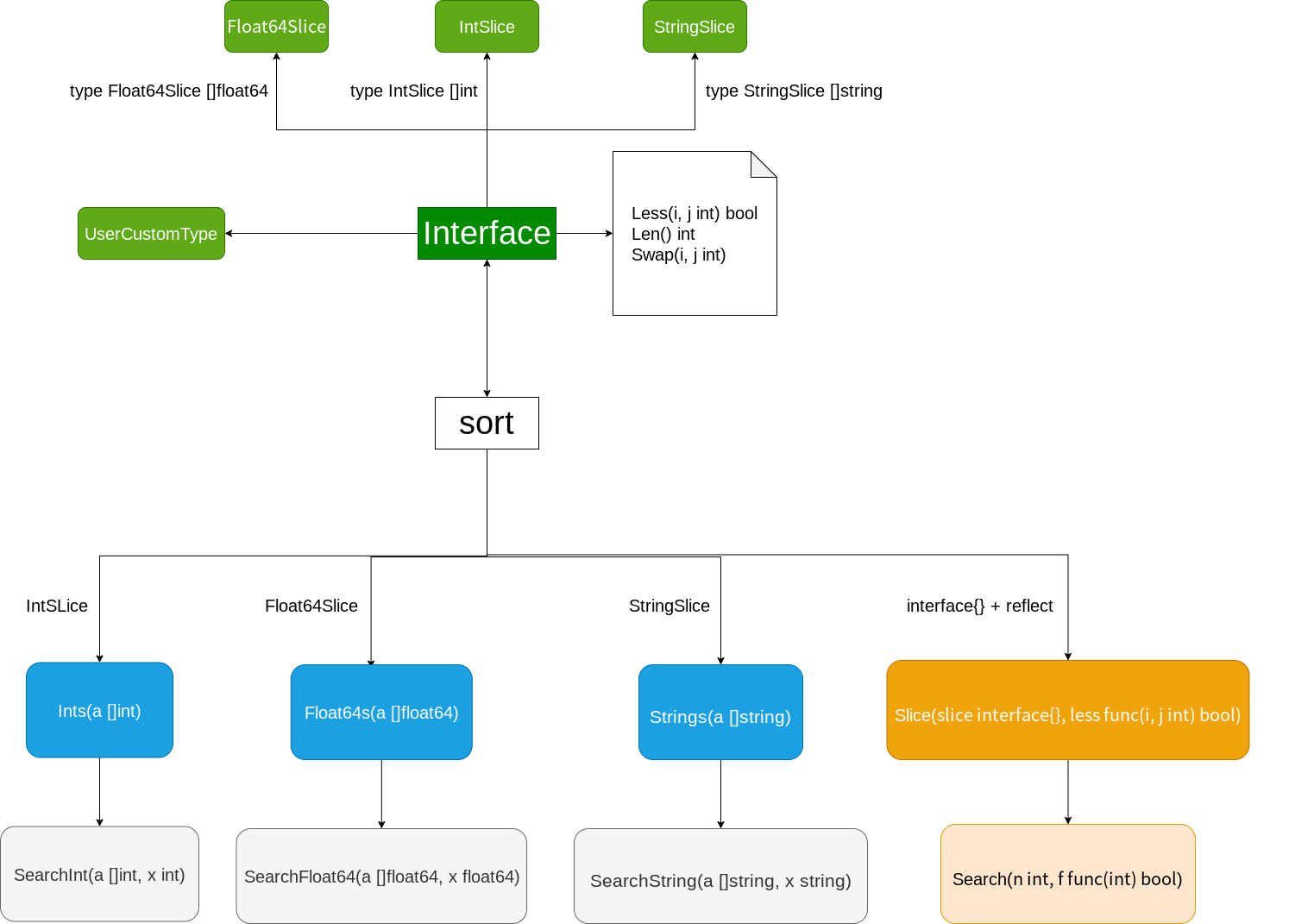## 沒有泛型的世界

### 暴力窮舉

``````float a = logf(2.0f);
double b = log(2.0);

typedef struct {
int *data;
unsigned int max_size;
} IntQueue;

typedef struct {
double *data;
unsigned int max_size;
} DoubleQueue;

IntQueue* NewIntQueue(unsigned int size)
{
IntQueue* q = (IntQueue*)malloc(sizeof(IntQueue));
if (q == NULL) {
return NULL;
}
q->max_size = size;
q->data = (int*)malloc(size * sizeof(int));
return q;
}

DoubleQueue* NewDoubleQueue(unsigned int size)
{
DoubleQueue* q = (DoubleQueue*)malloc(sizeof(DoubleQueue));
if (q == NULL) {
return NULL;
}
q->max_size = size;
q->data = (double*)malloc(size * sizeof(double));
return q;
}
``````

• 嚴重違反DRY（don’t repeat yourself），數據結構的修改和擴展極其困難
• 複製粘貼修改中可能會出現低級的人力錯誤，並且耗費精力
• 最關鍵的一點，我們不可能針對所有類型去寫出特定的演算法，因為這些類型的數量少則5,6種，多則上不封頂。

• 保證了類型安全，任何類型問題都能在編譯期暴露
• 更靈活，對於某些特定類型我們還可以做出非常細緻的優化工作（比如對於bool類型我們可以使用unsigned int這個一般來說4位元組大小的類型存放32個bool值，而不是用32個bool變數消耗32位元組記憶體）

### 依靠通用引用類型

``````void *ptr = NULL;
ptr = (void*)"hello";
int a = 100;
ptr = (void*)&a;
``````

c語言允許非函數指針的數據類型指針轉換為`void *`，因此我們可以用它來囊括幾乎所有的數據（函數除外）。

``````typedef struct {
void *data;
unsigned int max_size;
} Queue;

Queue* NewQueue(unsigned int size)
{
Queue* q = (Queue*)malloc(sizeof(Queue));
if (q == NULL) {
return NULL;
}
q->max_size = size;
q->data = // 這裡填什麼呢？
}
``````

``````typedef struct {
unsigned int max_size;
unsigned int current;
void **data;
} Queue;

Queue* NewQueue(unsigned int size)
{
Queue* q = (Queue*)malloc(sizeof(Queue));
if (q == NULL) {
return NULL;
}
q->max_size = size;
q->size = 0;
q->data = (void **)malloc(size*sizeof(void*));
}

bool QueuePush(Queue* q, void* value)
{
if (q == NULL || value == NULL || q->current == q->max_size-1) {
return false;
}

q->data[q->current++] = value;
return true;
}
``````

It works! 但是我們需要隊列中的類型有特定操作呢？把操作抽象形成函數再傳遞給隊列的方法就行了，可以參考c的qsort和bsearch：

``````#include <sdtlib.h>

void qsort(void *base, size_t nmemb, size_t size,
int (*compar)(const void *, const void *));

void *bsearch(const void *key, const void *base,
size_t nmemb, size_t size,
int (*compar)(const void *, const void *));
``````

``````typedef struct node {
int val;
struct node *next;
} node_t;

void enqueue(node_t **head, int val) {
node_t *new_node = malloc(sizeof(node_t));
if (!new_node) return;

new_node->val = val;

}
``````

• 首先是類型擦除的同時沒有任何類型檢測的手段，因此類型安全無從保證，比如存進去的可以是`int`，取出來的時候你可以轉換成`char*`，程式不會給出任何警告，等你準備從這個`char*`里取出某個位置上的字元的時候就會引發未定義行為，從而出現許許多多奇形怪狀的bug
• 只能存指針類型
• 如何確定隊列里存儲數據的所有權？交給隊列管理會增加隊列實現的複雜性，不交給隊列管理就需要手動追蹤N個對象的生命周期，心智負擔很沉重，並且如果我們是存入的局部變數的指針，那麼交給隊列管理就一定會導致free出現未定義行為，從程式碼層面我們是幾乎不能區分一個指針是不是真的指向了堆上的內容的
• 依舊不能避免書寫類型程式碼，首先使用數據時要從`void*`轉換為對應類型，其次我們需要書寫如qsort例子里那樣的幫助函數。

### 動態類型語言的特例

``````int a = 0;
const char *str = "hello generic";
double values[] = {1., 2., 3.};
``````

``````name = 'apocelipes'
name = 12345
``````

name究竟是什麼類型的變數？不知道，因為name實際上可以賦值任意的數據，我們只能在運行時的某個點做類型檢測，然後斷言name是xxx類型的，然而過了這個時間點之後name還可以賦值一個完全不同類型的數據。

``````class Queue:
def __init__(self):
self.data = []

def push(self, value):
self.data.append()

def pop(self):
self.data.pop()

def take(self, index):
return self.data[index]
``````

``````def transform(self):
for i in range(len(self.data)):
self.data[i] = self.data[i].upper()
``````

• 寫出了類型相關的程式碼，和我們本意上想要實現類型無關的程式碼結構相衝突
• 限定了演算法只能由幾種數據類型使用，但事實上有無限多的類型可以實現upper方法，然而我們不能在類型檢查里一一列舉他們，從而導致了我們的通用演算法變為了限定演算法。

## 動靜結合

### 使用interface模擬泛型

interface可以接受任何滿足要求的類型的數據，並且具有運行時的類型檢查。雙保險很大程度上提升了程式碼的安全性。

``````package list // import "container/list"

Package list implements a doubly linked list.

To iterate over a list (where l is a *List):

for e := l.Front(); e != nil; e = e.Next() {
// do something with e.Value
}

type Element struct{ ... }
type List struct{ ... }
func New() *List

type Element struct {

// The value stored with this element.
Value interface{}
// Has unexported fields.
}
Element is an element of a linked list.

func (e *Element) Next() *Element
func (e *Element) Prev() *Element

type List struct {
// Has unexported fields.
}
List represents a doubly linked list. The zero value for List is an empty

func New() *List
func (l *List) Back() *Element
func (l *List) Front() *Element
func (l *List) Init() *List
func (l *List) InsertAfter(v interface{}, mark *Element) *Element
func (l *List) InsertBefore(v interface{}, mark *Element) *Element
func (l *List) Len() int
func (l *List) MoveAfter(e, mark *Element)
func (l *List) MoveBefore(e, mark *Element)
func (l *List) MoveToBack(e *Element)
func (l *List) MoveToFront(e *Element)
func (l *List) PushBack(v interface{}) *Element
func (l *List) PushBackList(other *List)
...
``````

``````func IndexOfInterface(arr []interface{}, value interface{}) int {
for i, v := range arr {
if v == value {
return i
}
}

return -1
}
``````

### interface會進行嚴格的類型檢查

``````func ExampleIndexOfInterface() {
arr := []interface{}{uint(1),uint(2),uint(3),uint(4),uint(5)}
fmt.Println(IndexOfInterface(arr, 5))
fmt.Println(IndexOfInterface(arr, uint(5)))
// Output:
// -1
// 4
}
``````

``````type Comparator interface {
Compare(v interface{}) bool
}

func IndexOfComparator(arr []Comparator, value Comparator) int {
for i,v := range arr {
if v.Compare(value) {
return i
}
}
return -1
}
``````

### 內置類型何去何從

``````cannot use arr (type []interface {}) as type []Comparator in argument to IndexOfComparator
``````

``````type MyUint uint

func (u MyUint) Compare(v interface{}) bool {
value := v.(MyUint)
return u == value
}

arr2 := []Comparator{MyUint(1),MyUint(2),MyUint(3),MyUint(4),MyUint(5)}
fmt.Println(IndexOfComparator(arr2, MyUint(5)))
``````

### 性能陷阱

``````func IndexOfByReflect(arr interface{}, value interface{}) int {
arrValue := reflect.ValueOf(arr)
length := arrValue.Len()
for i := 0; i < length; i++ {
if arrValue.Index(i).Interface() == value {
return i
}
}
return -1
}

func IndexOfInterface(arr []interface{}, value interface{}) int {
for i, v := range arr {
if v == value {
return i
}
}

return -1
}

func IndexOfInterfacePacking(value interface{}, arr ...interface{}) int {
for i, v := range arr {
if v == value {
return i
}
}

return -1
}
``````

``````const ArrLength = 500
var _arr []interface{}
var _uintArr []uint

func init() {
_arr = make([]interface{}, ArrLength)
_uintArr = make([]uint, ArrLength)
for i := 0; i < ArrLength - 1; i++ {
_uintArr[i] = uint(rand.Int() % 10 + 2)
_arr[i] = _uintArr[i]
}
_arr[ArrLength - 1] = uint(1)
_uintArr[ArrLength - 1] = uint(1)
}

func BenchmarkIndexOfInterface(b *testing.B) {
for i := 0; i < b.N; i++ {
IndexOfInterface(_arr, uint(1))
}
}

func BenchmarkIndexOfInterfacePacking(b *testing.B) {
for i := 0; i < b.N; i++ {
IndexOfInterfacePacking(uint(1), _arr...)
}
}

func indexOfUint(arr []uint, value uint) int {
for i,v := range arr {
if v == value {
return i
}
}

return -1
}

func BenchmarkIndexOfUint(b *testing.B) {
for i := 0; i < b.N; i++ {
indexOfUint(_uintArr, uint(1))
}
}

func BenchmarkIndexOfByReflectInterface(b *testing.B) {
for i := 0; i < b.N; i++ {
IndexOfByReflect(_arr, uint(1))
}
}

func BenchmarkIndexOfByReflectUint(b *testing.B) {
for i := 0; i < b.N; i++ {
IndexOfByReflect(_uintArr, uint(1))
}
}
``````### 複合類型的迷思

`interface{}`是介面，而`[]interface{}`只是一個普通的slice。複合類型中的介面是不存在協變的。所以下面的程式碼是有問題的：

``````func work(arr []interface{}) {}

ss := []string{"hello", "golang"}
work(ss)
``````sort為了能處理slice，不得不包裝了常見的基本類型的slice，為了兼容自定義類型包里提供了`Interface`，需要你自己對自定義類型的slice進行包裝。

### 最後也是最重要的

``````var i interface{}
i = 1
s := i.(string)
``````

## 泛型帶來的影響，以及拯救

### 徹底從沒有泛型的泥沼中解放

``````package main

import (
"fmt"
)

func IndexOf[T comparable](arr []T, value T) int {
for i, v := range arr {
if v == value {
return i
}
}

return -1
}

func main() {
q := []uint{1,2,3,4,5}
fmt.Println(IndexOf(q, 5))
}
``````

`comparable`是go2提供的內置設施，代表所有可比較類型，你可以在這裡運行上面的測試程式碼。

``````func isEqual[T comparable](a,b []T) bool {
if len(a) != len(b) {
return false;
}

for i := range a {
if a[i] != b[i] {
return false
}
}

return true
}
``````

• 真正的類型安全，像`isEqual([]int, []string)`這樣的程式碼在編譯時就會被發現並被我們修正
• 雖然泛型也不支援協變，但slice等複合類型只要符合參數推導的規則就能被使用，限制更少
• 沒有了介面和反射，性能自不必說，編譯期就能確定變數類型的話還可以增加程式碼被優化的機會

### 泛型的代價

##### 參考

//colobu.com/2016/04/14/Golang-Generics-Proposal/